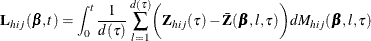#### Residuals

This section describes the computation of residuals (RESMART, RESDEV, RESSCH, and RESSCO in the OUTPUT statement). See the section Notation and Estimation for definition of notation that is used in this section. The residuals are calculated based on the TIES= option in the MODEL statement.

##### TIES=BRESLOW

This is the default option. Let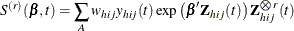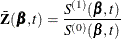where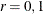; and A be the set of indices in the selected sample.

Further letThe martingale residual at t is defined as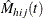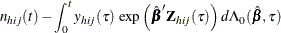Hereestimates the difference over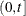between the observed number of events for the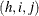observation unit and a conditional expected number of events. The quantityis referred to as the martingale residual for theobservation unit. For the Cox model with no time-dependent explanatory variables, the martingale residual for theunit with observation time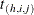and event status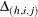is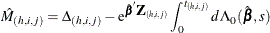The deviance residual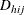for theobservation unit is a transformation of the corresponding martingale residuals,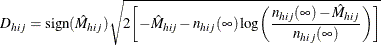The square root shrinks large negative martingale residuals, while the logarithmic transformation expands martingale residuals that are close to unity. As such, the deviance residuals are more symmetrically distributed around zero than the martingale residuals. For the Cox model, the deviance residual reduces to the form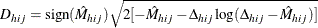The Schoenfeld (1982) residual vector is calculated on a per-event-time basis. At the kth event timeof theobservation unit, the Schoenfeld residual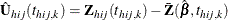is the difference between the observed covariate vector for theobservation unit and the average of the covariate vectors over the risk set at. Under the proportional hazards assumption, the Schoenfeld residuals have the sample path of a random walk; therefore, they are useful in assessing time trend or lack of proportionality.

The score process for thesubject at time t is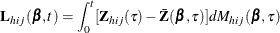The vector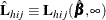is the score residual for theobservation unit.

The score residuals are a decomposition of the first partial derivative of the log likelihood. They are useful in assessing the influence of each subject on individual parameter estimates. They also play an important role in the computation of the variance estimators.

##### TIES=EFRON

For TIES=EFRON, the preceding computation is modified to comply with the Efron partial likelihood. For a given uncensored time t, let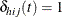if t is an event time for theobservation, and 0 otherwise. Let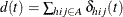, which is the number of observation units that have an event at t. For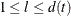, let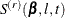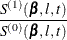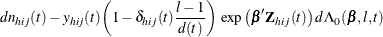where, and A are the set of indices in the selected sample.

The martingale residual at t for theobservation unit is defined as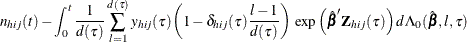Deviance residuals are computed by using the same transform on the corresponding martingale residuals as in TIES=BRESLOW.

The Schoenfeld residual vector for theobservation unit at event timeisThe score process for theobservation unit at time t is